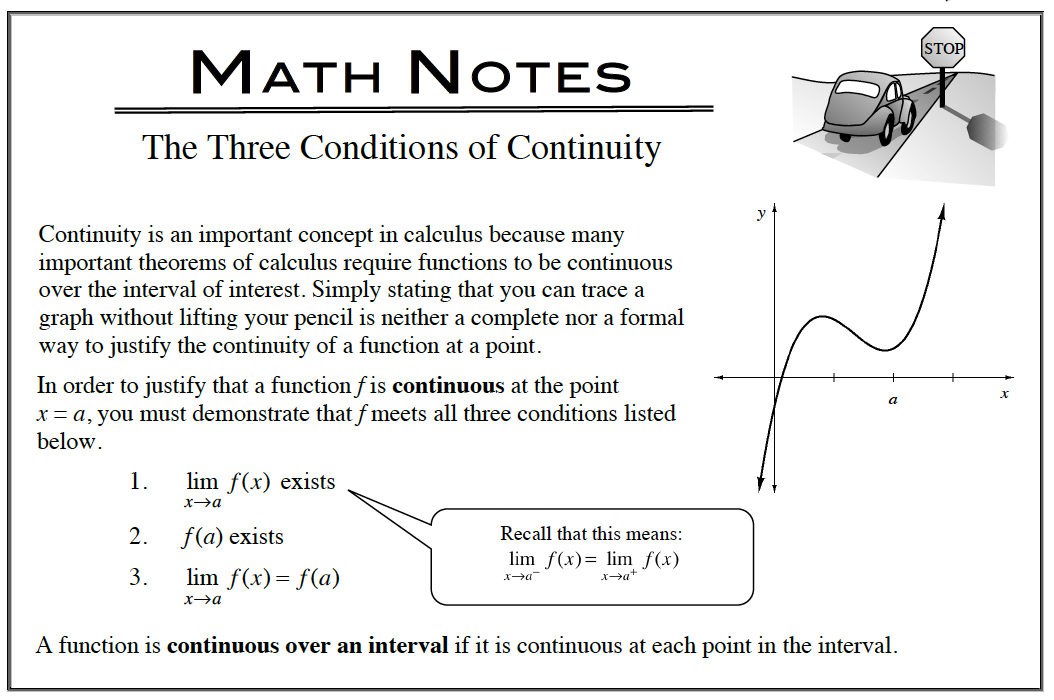### Home > APCALC > Chapter 6 > Lesson 6.5.1 > Problem6-178

6-178.

Given the piecewise-defined function below, what value of a will make this function continuous? 6-178 HW eTool (Desmos). Homework Help ✎

$f ( x ) = \left\{ \begin{array} { l l } { \sqrt { 1 - x } + 7 } & { \text { for } x > - 3 } \\ { - a x ^ { 3 } } & { \text { for } x \leq - 3 } \end{array} \right.$$\sqrt{1-(-3)}+7=-a(-3)^{3}$

Solve for a.

Use the eTool below to visualize the problem.
Click the link at right for the full version of the eTool: Calc 6-178 HW eTool